Next: Exercises Up: Rotating Reference Frames Previous: Coriolis Force

# Foucault Pendulum

Consider a pendulum consisting of a compact mass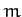suspended from a light cable of length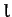in such a manner that the pendulum is free to oscillate in any plane whose normal is parallel to the Earth's surface. The mass is subject to three forces: first, the force of gravity, which is directed vertically downward (we are again ignoring centrifugal acceleration); second, the tensionin the cable, which is directed upward along the cable; and, third, the Coriolis force. It follows that the apparent equation of motion of the mass, in a frame of reference which co-rotates with the Earth, is [see Equation (414)](438)

Let us define our usual Cartesian coordinates (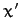,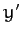,), and let the origin of our coordinate system correspond to the equilibrium position of the mass. If the pendulum cable is deflected from the downward vertical by a small angle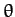then it is easily seen that,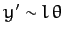, and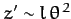. In other words, the change in height of the mass,, is negligible compared to its horizontal displacement. Hence, we can write, provided that. The tensionhas the vertical component, and the horizontal component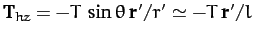, since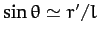--see Figure 27. Hence, the Cartesian equations of motion of the mass are written [cf., Equations (424)-(426)]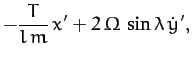(439)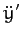(440)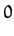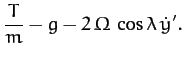(441)

To lowest order in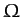(i.e., neglecting), the final equation, which is just vertical force balance, yields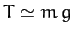. Hence, Equations (439) and (440) reduce to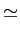(442)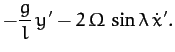(443)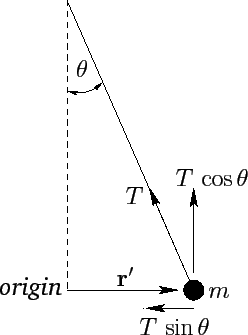Let(444)

Equations (442) and (443) can be combined to give a single complex equation for: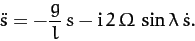(445)

Let us look for a sinusoidally oscillating solution of the form(446)

Here,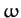is the (real) angular frequency of oscillation, and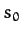is an arbitrary complex constant. Equations (445) and (446) yield the following quadratic equation for: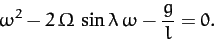(447)

The solutions are approximately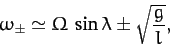(448)

where we have neglected terms involving. Hence, the general solution of (446) takes the form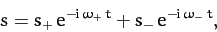(449)

where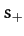andare two arbitrary complex constants.

Making the specific choice, whereis real, the above solution reduces to(450)

Now, it is clear from Equation (444) thatandare the real and imaginary parts of, respectively. Thus, it follows from the above that(451)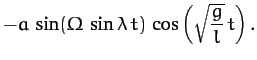(452)

These equations describe sinusoidal oscillations, in a plane whose normal is parallel to the Earth's surface, at the standard pendulum frequency. The Coriolis force, however, causes the plane of oscillation to slowly precess at the angular frequency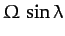. The period of the precession is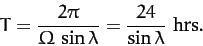(453)

For example, according to the above equations, the pendulum oscillates in the-direction (i.e., north/south) at, in the-direction (i.e., east/west) at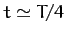, in the-direction again at, etc. The precession is clockwise (looking from above) in the northern hemisphere, and counter-clockwise in the southern hemisphere.

The precession of the plane of oscillation of a pendulum, due to the Coriolis force, is used in many museums and observatories to demonstrate that the Earth is rotating. This method of making the Earth's rotation manifest was first devised by Foucault in 1851.Next: Exercises Up: Rotating Reference Frames Previous: Coriolis Force
Richard Fitzpatrick 2011-03-31